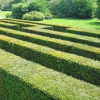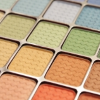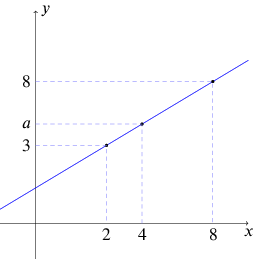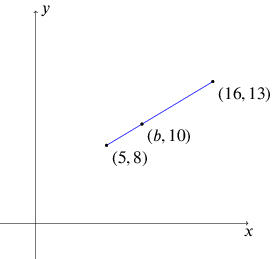#### You may also likeThis comes in two parts, with the first being less fiendish than the second. Itâ€™s great for practising both quadratics and laws of indices, and you can get a lot from making sure that you find all the solutions. For a real challenge (requiring a bit more knowledge), you could consider finding the complex solutions.### Discriminating

You're invited to decide whether statements about the number of solutions of a quadratic equation are always, sometimes or never true.This will encourage you to think about whether all quadratics can be factorised and to develop a better understanding of the effect that changing the coefficients has on the factorised form.

# Between

##### Age 16 to 18Challenge LevelThis resource is from Underground Mathematics.

1.  Here is a graph showing a straight line.  It is not drawn accurately.

The points $(2,3)$ and $(8,8)$ lie on the line.

Another point, $(4,a)$, also lies on the line.  Can you work out
the value of $a$?

How many different ways can you find to do this?2.  This time, the $x$-coordinate of one of the points is missing - can you work it out?Did all of your approaches from question 1 work, or did some not?
Or perhaps they worked with some modification?

Can you find any new ways to do this question which are
different from your methods for question 1?

3.  This time, you might find it helpful to draw your own sketches.

• The point $(7.3, c)$ lies on the straight line joining $(4.1, 37)$ and $(8.9, 63)$.  Find $c$.

• The point $(d, 47.5)$ lies on the straight line joining $(15.05, 42)$ and $(17.55, 56)$.  Find $d$.

• The point $(12, e)$ lies on the straight line joining $(8, 20)$ and $(17, 1)$.  Find $e$.

This is an Underground Mathematics resource.

Underground Mathematics is hosted by Cambridge Mathematics. The project was originally funded by a grant from the UK Department for Education to provide free web-based resources that support the teaching and learning of post-16 mathematics.

Visit the site at undergroundmathematics.org to find more resources, which also offer suggestions, solutions and teacher notes to help with their use in the classroom.